# Classification Model in Machine Learning

October 12, 2020

By  Roshini

`lang: en_US`

These days the term Machine Learning is used in almost every field and is said to be the future of technology. Classification is one of these most important aspects of where machine learning is used. These buzz words are not as tricky as they seem to be. Interested in knowing what it is about and how MATLAB supports this concept? In this blog, we shall see how we can learn the basics of practical machine learning methods for classification problems. For those looking for a step by step instruction on creating a classification model right from importing data to calculating accuracy, this would be the right place. We shall see how to do this with a task to distinguish the letters "J", "V" and "M" and classify them into their respective group.

## Data Used:

Different people were asked to write the letters "J", "V" and "M" on a tablet, and the handwritten letters were stored as individual text files. Each file contains four columns, each separated by a comma. The four columns are a timestamp, the horizontal location of the pen, the pen's vertical location, and the pressure of the pen. The timestamp is the number of milliseconds elapsed since the beginning of the data collection. The other variables are in normalized units, i.e., from 0 to 1. The main folder has three sub-folders, one for each J, V, and M.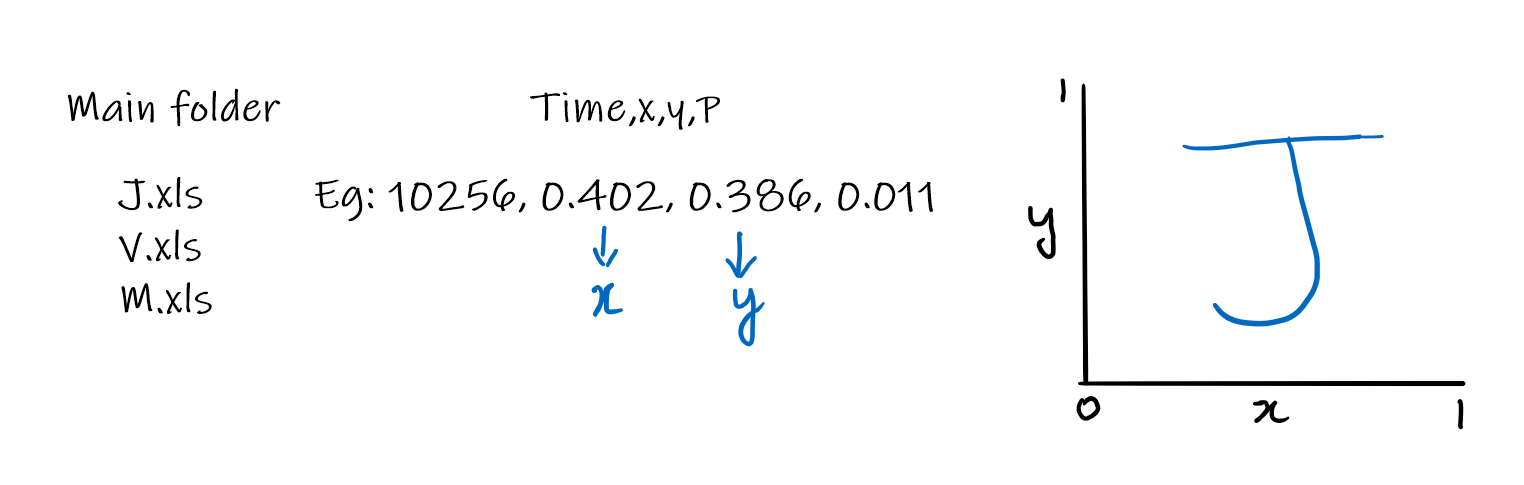## Import data:

We aim to create a model to classify an image as either letter J or V or M. Our first step towards this is importing the Handwriting data into MATLAB.

You can use the readtable function to import the tabular data from a spreadsheet or text file and store the result as a table.

This imports the data from the text file J.txt and stores it in a table called letter.

Our next step will be to extract the variable X from the table and the variable Y. You can use dot notation to get the column separately from a table. Then, use the plot function to plot them.

plot(letter.X ,letter.Y)

You can use the axis equal command to force the axes to preserve the data's aspect ratio. This will help in getting a more evident plot of the letter.

axis equal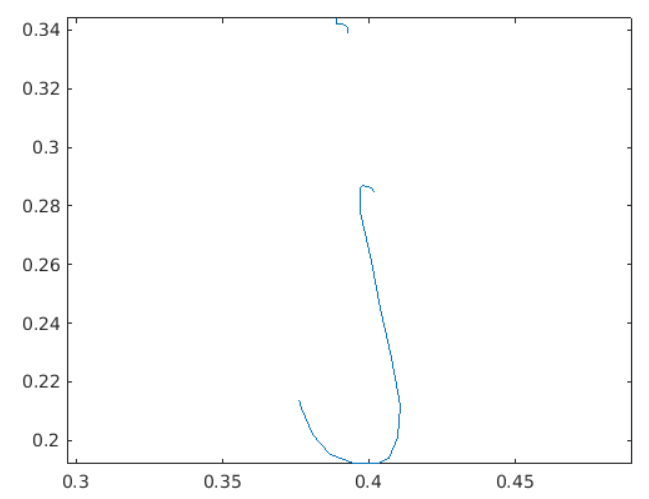This is the output after the command which shows the letter J.

Repeat the same importing and plotting tasks for the data in the file M.txt and V.txt.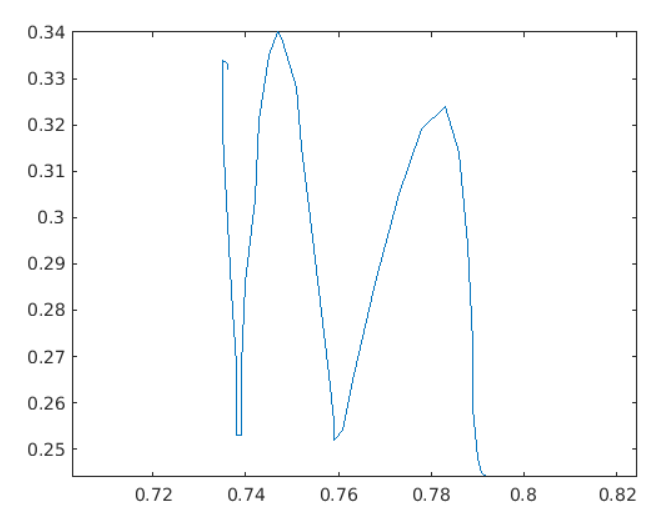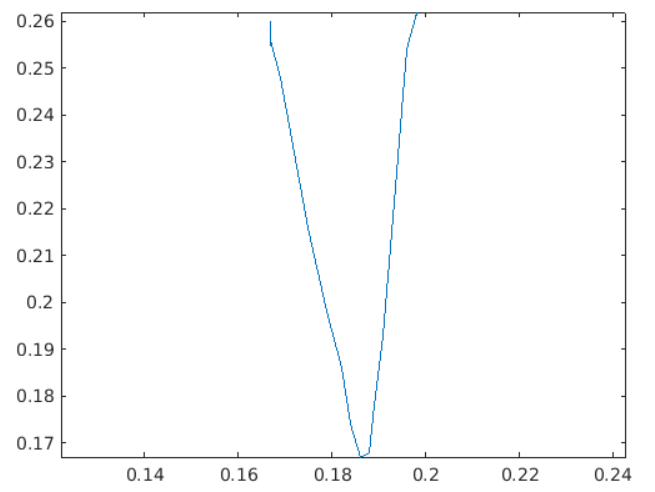## Process data:

### Correcting Units

The pen positions for the handwriting data are not measured in normalized units. Also, the tablet used to record the data is not square. This means a vertical distance of 1 corresponds to 10 inches, while the same horizontal distance corresponds to 15 inches. To correct this, the horizontal units should be adjusted to the range [0 1.5].You can use dot notation to extract, modify, and reassign variables in a table, just as you would with any variable. Multiply the values in the X variable of the table letter by the aspect ratio of 1.5.

letter.X = 1.5*letter.X;

The first picture is before changing the aspect ratio and the second picture is after changing the ratio during the pre-processing of data.

Shift the table letter's Time variable to start at 0 by subtracting the first value from all elements. Divide the result by 1000 to convert to seconds.

letter.Time = (letter.Time - letter.Time(1))/1000;

## Extract features:

### Calculating features:

What property of these letters can we use to distinguish a J from an M or a V? This property that we will find is different for each letter is called a feature. A feature is simply a value calculated from the signal, such as its duration.

1. For the letters J and M, a simple feature might be the aspect ratio (the height of the letter relative to the width). J is likely to be taller and will have less width, whereas an M is likely to be square.
1. Compared to J and M, a V is quick to write, so the signal's duration might also be a feature.

Calculate the time taken to write the letter by extracting the last value of the letter. Time and storing the result in a variable called dur.

dur = letter.Time(end)

Use the range function to calculate the letter's aspect ratio by dividing the range of values of letter.Y by the range of values of letter.X. Assign the result to a variable called aratio.

The range function returns the range of values in an array. That is, range(x) is equal to max(x)-min(x).

aratio = range(letter.Y)/range(letter.X)

Change the file name and rerun the script to calculate the same two features for the letters in J.txt and V.txt.

Output

Output for J:

dur =

0.4020

aratio =

3.8205

Output for M:

dur =

0.4560

aratio =

1.1064

Output for V:

dur =

0.2760

aratio =

1.6042

## View Features

The MAT-file featuredata.mat contains a table of the extracted features for these three letters written by a variety of people. The table "features" has three variables: AspectRatio and Duration (the two features calculated in the previous section), and Character (the known letter).

The gscatter function makes a grouped scatter plot; a scatter plot where the points are coloured according to a grouping variable. Use the gscatter function to create the same as a scatter plot but coloured according to the letter, stored in the Character variable of the table features.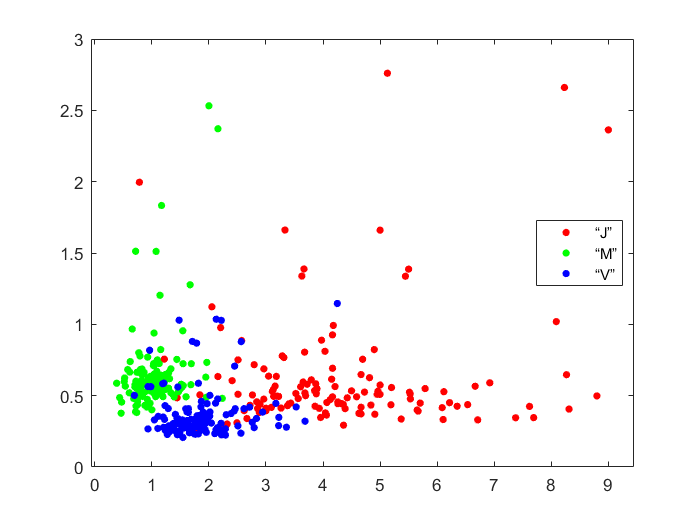## Build a model:

### What is a classification model?

In machine learning, classification refers to a predictive modelling problem where a class label is predicted for a given example of input data. Each region is assigned one of the output classes.

There is no single absolute "correct" way to partition the plane into the classes J, M, and V. Different classification algorithms result in different partitions.

An easy way to classify an observation is to use the same class as the nearest known examples. This is called a k-nearest neighbor(kNN) model. The kNN model works on the principle same class points are together. It would calculate the distance between 2 points in a graph, and if the distance is high, it will classify it as a different group.

Use the fitcknn function to fit a model to the data stored in features. The known classes are stored in the variable called Character. Store the resulting model in a variable called knnmodel.

`knnmodel = fitcknn(features,`"Character")

Output

knnmodel =

ClassificationKNN

PredictorNames: {'AspectRatio' 'Duration'}

ResponseName: 'Character'

CategoricalPredictors: []

ClassNames: {'“J”' '“M”' '“V”'}

ScoreTransform: 'none'

NumObservations: 394

Distance: 'euclidean'

NumNeighbors: 1

Having built a model from the data, you can use it to classify new observations. Now, this new data is the test data used to test the classification model. The testdata contains observations for which the correct class is known. This gives a way to test your model by comparing the classes predicted by the model with the true classes. The predict function will ignore the Character variable when making predictions from the model. Use the predict function with the trained model knnmodel to classify the letters in the table testdata. Store the predictions in a variable called predictions.

predictions = predict(knnmodel,testdata);

predictions=cell2mat(predictions)

Output

predictions =

20×3 char array

'“J”'

'“V”'

'“V”'

'“M”'

“J”'

'“J”'

'“V”'

'“J”'

'“M”'

'“M”'

'“J”'

'“J”'

'“J”'

'“J”'

'“M”'

'“M”'

'“V”'

'“J”'

'“V”'

'"J"'

By default, fitcknn fits a kNN model with k = 1. That is, the model uses just the single closest known example to classify a given observation. You can make the model more accurate in the testing data by increasing the value of k.

## Evaluate the model

How can we know how efficient is the kNN model, and how good is this model in classification? The table testdata includes the known class for the test observations. You can compare the known classes to the kNN model's predictions to see how well the model performs on new data.

Use the == operator to compare predictions to the known classes (stored in the variable Character in the table testdata). Store the result in a variable called iscorrect.

iscorrect=predictions==char(testdata.Character);

iscorrect=iscorrect(:,2)

Output

iscorrect =

20×1 logical array

1

1

1

1

0

0

1

1

1

1

1

1

1

1

1

1

0

0

1

1

Calculate the proportion of correct predictions by dividing the number of correct predictions by the total number of predictions. You can use the sum function to determine the number of correct predictions and the numel function to determine the total number of predictions.

accuracy = sum(iscorrect)*100/20

Output:

accuracy =

80

## Applications

With these instructions on creating a model, it is effortless to perform classification tasks in MATLAB, and they can also be extended to various other real-life applications such as:

1. Medical analysis: Classify the severity of a disease using the scans
2. Factories: Classify the defects on a final product
3. Traffic: Classify the vehicles on a road as 2-wheeler or 4-wheeler, etc
4. Banks: Classify the people who will be able to repay the loan and who won't

Ready to take your MATLAB skills to the next level? Look no further! At MATLAB Helper, we've got you covered. From free community support to expert help and training, we've got all the resources you need to become a pro in no time. If you have any questions or queries, don't hesitate to reach out to us. Simply post a comment below or send us an email at [email protected].

And don't forget to connect with us on LinkedIn, Facebook, and Subscribe to our YouTube Channel! We're always sharing helpful tips and updates, so you can stay up-to-date on everything related to MATLAB. Plus, if you spot any bugs or errors on our website, just let us know and we'll make sure to fix it ASAP.

Ready to get started? Book your expert help with Research Assistance plan today and get personalized assistance tailored to your needs. Or, if you're looking for more comprehensive training, join one of our training modules and get hands-on experience with the latest techniques and technologies. The choice is yours – start learning and growing with MATLAB Helper today!

Education is our future. MATLAB is our feature. Happy MATLABing!

#### Roshini

An Electronics and Communication engineering student. Passionate about learning and sharing with others.

#### MATLAB Helper ®

Use Website Chat or WhatsApp at +91-8104622179